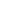艾天动力企业云邮全面升级

• 公司新闻 2018-05-29 分享新闻到：
<返回列表

1、稳定集群架构

2、无限存储空间

3、群组分权管理

4、强力反垃圾

5、超强企业网盘

6、丰富个性功能

 全球云邮功能 云邮基础版 云邮专业版 旧免费邮局 旧收费邮局 邮箱配置 邮箱用户数 5用户 5用户 - ∞ 5用户 5用户 - ∞ 邮箱总容量 5GB 无限 500MB 1GB/10GB x 用户数 文件中转站容量 1GB 10GB × × 个人网盘容量 × 5GB 500MB 500MB 企业网盘容量 × 1GB x 用户数 × × 邮箱管理功能 创建部门/群组 × √ × × 设置分级管理员 × √ × × 邮件审核 × √ × × 邮箱搬家 √ √ × × 坐席工作统计 × √ × × 自定义欢迎邮件 √ √ × × 添加置顶公告 √ √ × × 添加企业公告 √ √ √ √ 公共通讯录 √ √ √ √ 自定义公司名称 √ √ √ √ 自定义logo √ √ √ √ 添加别名 √ √ √ √ 黑名单管理 √ √ √ √ 白名单管理 √ √ √ √ 邮箱日志 √ √ √ √ 个人管理功能 日程计划 × √ √ √ 更换皮肤 √ √ √ √ 中英文切换 √ √ √ √ 时区设置 √ √ √ √ 我的日程通知 × √ √ √ 分享的日程通知 × √ × × 新邮件到达微信通知 √ √ × × 新邮件到达浏览器通知 √ √ × × 异地登录通知 √ √ × × 群邮件微信通知 √ √ × × 自定义时间段微信通知 √ √ × × 本地电脑挂载网盘 × √ × × 宽屏窄屏切换 √ √ × × 假期自动回复设置 √ √ × √ 自动转发设置 √ √ × √ 登录二次验证 √ √ × × 设置头像 √ √ × × 多别名账户管理 √ √ × × 微信扫码登录 √ √ × × 键盘快捷键操作 √ √ × × 新建便签 √ √ √ √ 设置便签提醒 × √ × × 签名设置 √ √ √ √ 邮件收发功能 在线上传附件大小 30MB 30MB 20MB 20MB SMTP服务 √ √ √ √ POP3服务 √ √ √ √ IMAP服务 √ √ × × SSL加密 √ √ × × 收信规则设置 × √ × × 邮件模板 √ √ × × 邮件模板分类 √ √ × × 邮件撤回 √ √ × × 拖拽上传 √ √ × × 断点续传 √ √ × × 超大附件 √ √ × × 收件人自动输入 √ √ × × 发送状态查询 √ √ × × 已读未读查询 √ √ × × 附件预览 √ √ × × 邮件打包下载 √ √ × × 一键归档 √ √ × × 全文搜索 √ √ × × 邮件会话 √ √ × × 来往邮件 √ √ × × 邮件备注 √ √ × × 子邮件夹 √ √ × × 多标签页 √ √ × × 分别发送 √ √ × × 客户端发信保存到已发送 √ √ × × 自动保存最近联系人 √ √ × × 设置重要联系人 √ √ √ √ 添加自定义文件夹 √ √ √ √ 添加自定义邮件标签 √ √ √ √ 添加第三方邮箱 √ √ √ √ 设置星标邮件 √ √ √ √ 企业内部发群邮件 √ √ √ √ 设置定时邮件 √ √ √ √ 设置紧急邮件 √ √ √ √ 设置需要回执 √ √ √ √ 邮件搜索 √ √ √ √ 注：收费邮箱购买用户数最少5个，最多无上限。免费邮箱免费时间为1年。各功能详情请联系我司在线客服人员咨询。

→立即登录演示体验

1. 登录用户管中心,在左侧菜单栏中依次选则”企业邮局管理”
2. 在右侧邮局列表中点击”升级”按钮,根据提示，一键免费升级到有云邮，老用户首年免费升级使用，第二年续费相较于旧邮箱价格略有提升。

更多阅读

五个步骤轻松搞定网站建设流程粤公网安备 44040202000180号7X24小时服务热线

0756-2286686

0756-2222010

0756-2286686

0756-2222010

0756-2222010

微信公众号微信号：aitiandongli